Next: 16. Syntax: Parsing and Up: 2. User's Guide Previous: 14. Macetes

Subsections

# 15.1 Motivation

The iota or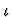constructor is a definite description operator for objects of kind. Given a variable x of sort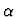of kindand a unary predicate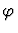,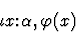denotes the unique x that satisfiesif there is such an x and is undefined otherwise. For example,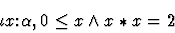denotes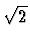but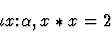is undefined.

The iota constructor is very useful for specifying functions, especially partial functions. For example, ordinary division of sort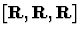(which is undefined whenever its second argument is 0) can be defined from the times function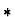by the lambda-expression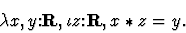Of course, this kind of definite description operator is only possible in a logic that admits undefined expressions.

# 15.2 Reasoning with Iota

An iota-expression is any expression which begins with the iota constructor (without any abbreviations by quasi-constructors). Proving a sequent with an assertion containing an iota-expression can be tricky. The key idea is to reduce the sequent to a new sequent with one less iota-expression. This is called eliminating iota.'' There are two commands and one macete for eliminating iota, each of which is discussed below in a separate subsection.

## 15.2.1 eliminate-defined-iota-expression

This command, which is described in Chapter 18, is the best iota-elimination tool in IMPS. Generally, you should use it whenever you want to eliminate an iota-expression whose definedness is implied by the sequent's context. It can also be used effectively in some cases on an iota-expression E whose definedness is not implied by the sequent's context. This is done by applying case-split with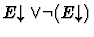, followed by applying eliminate-defined-iota-expression on the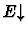case.

The eliminate-defined-iota-expression command is multi-inference; it adds about 20 new sequent nodes to a deduction graph.

## 15.2.2 eliminate-iota

This command is also described in Chapter 18. It is a single-inference command that is applicable to just atomic and negated atomic formulas. You should use it when you want to eliminate an iota-expression whose definedness is not implied by the sequent's context.

## 15.2.3 eliminate-iota-macete

This is a compound macete with built-in abstraction specified by:

   (def-compound-macete ELIMINATE-IOTA-MACETE
(sequential
iota-abstraction-macete
(series
tr%defined-iota-expression-elimination
tr%negated-defined-iota-expression-elimination
tr%left-iota-expression-equation-elimination
tr%right-iota-expression-equation-elimination)
beta-reduce))

The four elimination theorems are in the file \$IMPS/theories/generic-theories/iota.t. You should try eliminate-iota-macete when the assertion has one of the following forms:
1.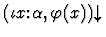2.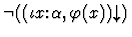3.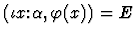4.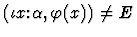5.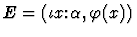6.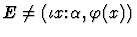7.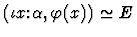8.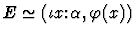For forms (1)-(6), the macete can be applied immediately, but for forms (7) and (8), the command insistent-direct-inference must be applied first. Beware that, for various reasons, eliminate-iota-macete may fail to do anything, in which case you should use one of the two iota-elimination commands.

# 15.3 Hints and Cautions

1.
Suppose E is an expression of sort. Sometimes it is convenient to have a new expression E' of sort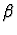(where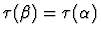but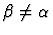) such that Eand E' have the same denotation. Such an expression can be easily constructed from E andusing iota: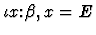is quasi-equal to E and is of sort. For example,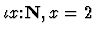denotes the natural number 2. It is important to mention here that the IMPS simplifier reduces an iota-expression of the formto the conditional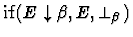, and further to E or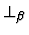if it can decide the formula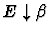.

2.
Suppose f is a function constant defined to be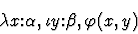such that the following existence implies uniqueness'' theorem holds for: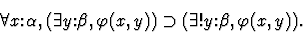By virtue of this theorem, there is an iota-free characterization'' of f,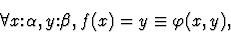which enables one to prove a formula f(a)=b by showing only existence--without employing any of the aforementioned tools for eliminating iota.

As an example, consider the limit operator lim%rr on sequences of reals defined by:

   (def-constant LIM%RR
"lambda(s:[zz,rr], iota(x:rr, forall(eps:rr,
0<eps
implies
forsome(n:zz, forall(p:zz,
n<=p implies abs(x-s(p))<=eps)))))"
(theory h-o-real-arithmetic))

Since a sequence always has at most one limit point, there is an iota-free characterization of lim%rr:
   (def-theorem CHARACTERIZATION-OF-REAL-LIMIT
"forall(s:[zz,rr],
x:rr,lim%rr(s)=x
iff
forall(eps:rr,
0<eps
implies
forsome(n:zz, forall(p:zz,
n<=p implies abs(x-s(p))<=eps))))"
(theory h-o-real-arithmetic))


3.
It is rarely a good idea to unfold a constant defined as an iota-expression, if it has an iota-free characterization. Instead, the following steps are suggested. For concreteness, let us assume the constant we are unfolding is a function constant f as in the previous item. Our aim is to reduce all occurrences of f to occurrences of the form f(a)=b,
• If all occurrences in the formula are of the form f(a)=b then apply the iota-free characterization of f as a macete.
• Otherwise, for every occurrence f(a), where a is a free variable, cut with the formula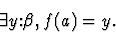Each application of cut adds two new sequent nodes to the deduction graph:
• The cut major premise, which is the original sequent plus the cut formula as another assumption.
• The cut minor premise, which has the cut formula as the assertion. The minor premise can be proved by instantiating y with f(a). To proceed with this part of the proof you will need to establish the definedness of f(a).
For a precise description of the sequent nodes added by cut, see the documentation on the command cut-with-single-formula.
• To deal with the major premise, do an antecedent inference on the cut formula to remove its existential quantifier. This yields a new goal with an assumption of the form f(a)=v.
• Backchain repeatedly on f(a)=v. This replaces all occurrences of f(a) with v.
• Incorporate the antecedent f(a)=v and apply the iota-free characterization of f as a macete. This should replace all such occurrences with some condition not involving f, and more importantly, not involving iota.

4.
The iota-p constructor is a definite description operator for objects of kind. It has a different semantics than iota: if there is no unique x of sortsatisfying a unary predicate, then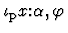is defined (like all expressions of kind) but has the value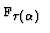. There is currently no support in IMPS for reasoning about the iota-p. This should not cause you any concern because iota-p has little practical value.Next: 16. Syntax: Parsing and Up: 2. User's Guide Previous: 14. Macetes